Courses

# Energy Dissipation in Structural Materials Civil Engineering (CE) Notes | EduRev

## Civil Engineering (CE) : Energy Dissipation in Structural Materials Civil Engineering (CE) Notes | EduRev

The document Energy Dissipation in Structural Materials Civil Engineering (CE) Notes | EduRev is a part of Civil Engineering (CE) category.
All you need of Civil Engineering (CE) at this link: Civil Engineering (CE)

Introduction
• Selection of structural materials corresponding to high inherent energy dissipation or damping depends on three factors

• material properties
• geometric property of the structural member and

• Material properties are connected with the system parameters as follows:
• Damping capacity of structural materials may depend on the following mechanisms

• dislocation movement: this occurs due to the presence of slip planes in crystalline materials;
• grain boundary slip: movement of one grain over the other causes energy dissipation;
• magnetoelastic effect: due to interaction between magnetization and strain of a magnetic material;
• thermoelastic effect: due to interaction between thermal and mechanical deformation;
• localized plastic strain - presence of defects like shear bands can entangle dislocations preventing the crystal from sliding. This may also create energy dissipation.

Stress Dependence of Energy Dissipation
The stress-strain plot of a structural material (metals and metallic alloys are considered here) under harmonic loading and low stress level may be plotted as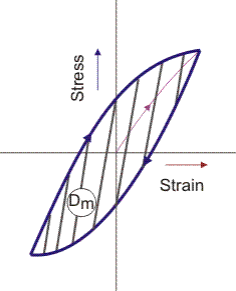Figure 9.1: Stress strain plot

• The energy dissipated per unit volume of a structural material per unit cycle is given by the area of the hysteresis loop (also known as mechanical hysteresis loop).
• This is generally denoted as Dm (dissipated energy per m3 per cycle)
• The energy dissipated per unit volume per cycle, Dm is related to the applied stress as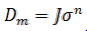where J is the damping constant and n the damping index.

• At a very low stress level, n=2 and the stress diagram becomes elliptic instead of showing pointed tip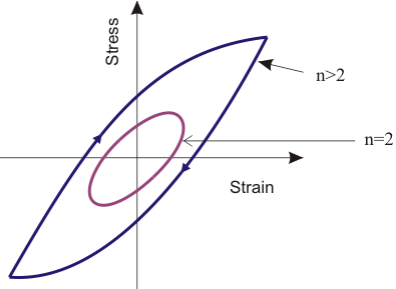Figure 9.2 : Energy dissipation at different stress level

Generally n varies from 2 to 3. For, higher values of n a modified relationship is used as follows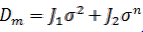whereJ1, J2 are the damping constants.

For multi axial loading of a structural member, The Dm is given by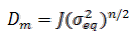Note that the uniaxial stress σ is replaced by equivalent stress,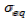.is denoted ass1, s2, s3 are the principal stress amplitudes. λ1, usually is very small.
The material loss factor ηm could be expressed in terms of Dm as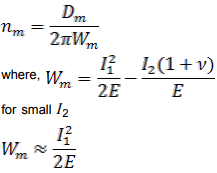The total loss factor of a composite specimen ηs can be obtained as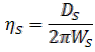where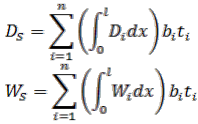bi - width of the i-th layer
ti - thickness of i-th layer

Table below shows the list of density, Young's modulus, and the order of loss factor of a few common structural materials.

Table 9.1: Mechanical properties of important structural materials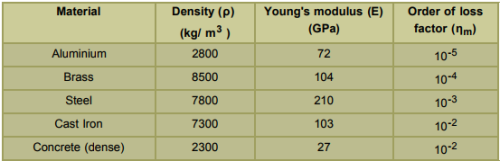Offer running on EduRev: Apply code STAYHOME200 to get INR 200 off on our premium plan EduRev Infinity!

,

,

,

,

,

,

,

,

,

,

,

,

,

,

,

,

,

,

,

,

,

;